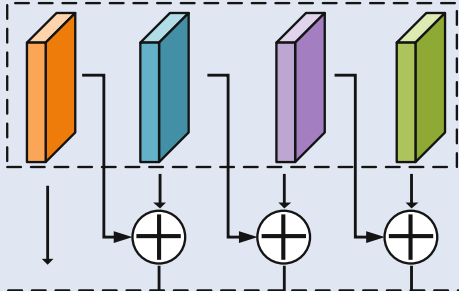# Element wise addition with temporary tensors

I am trying to implement the following part of a modelthis is the code I wrote

``````            x1, x2, x3, x4 = level(x1, x2, x3, x4)

x2 = tempx2

x3 = tempx3

x4 = tempx4
``````

do you find it right,
I am a beginner to pytorch, but I think the “temp” tensors I create could be a source to memory leakage because this calculation is inside a loop

It can’t be the case, as python implements a reference count based garbage-collection. i.e., When the reference count of an object reaches 0, reference counting garbage collection algorithm cleans up the object.

In python, for simplicity, you can do as below:

``````x2, x3, x4 = x1+x2, x2+x3, x3+x4
``````
1 Like